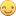## SAS VA 8.3 Display rule interval using Parameter

Hi,

I would like to use parameter for the display rule's interval instead of hard coded it because my interval may change depending on data. May I know how can I achieve this? Thanks

4 REPLIES 4

## Re: SAS VA 8.3 Display rule interval using Parameter

Hi!

One way is to create a calculated item that uses your parameter and then create the display rule on that calculated item.

//Fredrik

## Re: SAS VA 8.3 Display rule interval using Parameter

Hi Fredrike,

Thanks for you time on my question. But, I would like to have the parameter on the interval eg.(1 >=  <= 5 ; 5 > <= 10 ; 10 > <= 15), those number to parameter, understand that it is not possible but is there any other way to achieve this? Thanks

## Re: SAS VA 8.3 Display rule interval using Parameter

Maybe i am way out here 🙂

but...

IF you create a calculated measure that use your parameter or parameters to create a status and then create a display rule on that status, something like this:

If [parameter] = '1 >=  <= 5' and (1 <= [value] <=5) then status = 1

else If [parameter] = '5 >=  <= 10' and (5 <= [value] <=10) then status = 1      ***** (or 2 if you want to have different display rules)

else If [parameter] = '10 >=  <= 15' and (10 <= [value] <=15) then status = 1      ***** (or 3 if you want to have different display rules)

Won't that work fo you?

//Fredrik

## Re: SAS VA 8.3 Display rule interval using Parameter

Hi Fredrike,

That was a good ideabut it still not achievable on GAUGE right?

Discussion stats
• 4 replies
• 494 views
• 0 likes
• 2 in conversation